# Prediction ellipse and prediction ellipsoid¶

Marcos Duarte

To describe the dispersion of data or to make a prediction for a sample, we can calculate a prediction interval or tolerance interval. For the specific case of a univariate random variable, see Confidence and prediction intervals. For a multivariate random variable, we need to calculate a prediction hyperellipsoid (Chew, 1966):

The 95% prediction hyperellipsoid is a prediction interval for a sample of a multivariate random variable such that there is 95% of probability that a new observation will lie inside the hyperellipsoid.

The 95% prediction interval (a p-dimensional hyperellipsoid) for a sample of a multivariate random variable with $p$ dimensions and size $n$ from a population with normal distribution is given by the following probabilistic bounds which holds 95% of the time (Chew, 1966):

$$(x_{n+1}-\bar{x})'\: S^{-1} (x_{n+1}-\bar{x}) \leq \frac{F_{(0.95,\: p,\: n-p)}(n-1)\:p\:(n+1)}{n\:(n-p)}$$

Where $x_{n+i}$ (with dimension $p$) is the new observation for which we want to calculate the prediction interval; $F$ is the F-distribution; $\bar{x}$ is the sample mean (with dimension $p$), and $S$ is the sample covariance matrix defined as:

$$S_{j,k} = \frac{1}{n-1}\sum_{i=1}^{n}(x_{ij}-\bar{x}_j)(x_{ik}-\bar{x}_k)$$

This is the formula for the prediction of the next single observation where the population mean and variance are unknown (we only have estimates of these parameters from the sample). This formula is also equivalent to the formula for calculation of a tolerance region (called Type 2 tolerance region by Chew (1966)):

The 95% tolerance hyperellipsoid is a tolerance region for a sample of a multivariate random variable such that the average or expected value of the proportion of the population contained in the hyperellipsoid is 95%.

In this context, we can refer to the calculated hyperellipsoid as a prediction or tolerance hyperellipsoid (but prediction and tolerance intervals are in general different concepts in statistics).

The function hyperellipsoid.py (code at the end of this text) calculates the prediction or tolerance hyperellipsoid, some related parameters, and plots the results for a given multivariate random variable. The function signature is:

hypervol, saxes, angles, p0, R = hyperellipsoid(P, y=None, z=None, pvalue=.95,
units=None, show=True, ax=None):


Before showing how to use this code, let's look a bit more at the statistical principles behind the estimation of a prediction or tolerance interval.

## Univariate variable¶

For $p$ = 1 (univariate case) the 95% prediction interval formula above reduces to:

$$\left|\frac{x_{n+1}-\bar{x}}{s}\right| \leq \sqrt{F_{(0.95,\: 1,\: n-1)}(1+1/n)}$$

Where $s$ is the square root of the sample covariance, the sample standard deviation.

And considering that $F_{(0.95,\: 1,\: n-1)}$ is equal to $T^2_{(0.975,\: n-1)}$, the square of the Student's t-distribution, finally we have:

$$\left|x_{n+1}-\bar{x}\right| \leq s\:T_{(0.975,\: n-1)}\sqrt{1+1/n}$$

Indeed, this results in the 95% prediction interval used for a sample of a univariate variable, see Confidence and prediction intervals.

For large $n$, according to the central limit theorem, we can use the fact that $T(\infty)=N$, where $N$ is the normal distribution, and the above statement can be simplified to:

$$\left|x_{n+1}-\bar{x}\right| \leq s\:N_{0.975}$$

$N_{0.975}$ is equal to 1.96, a commonly used value for calculating 95%-probability intervals.
Let's calculate the error of this approximation for different sample sizes.
First let's import the necessary Python libraries:

In :
# import the necessary libraries
import numpy as np
from scipy import stats

In :
F = lambda p, n: np.sqrt(stats.f.ppf(0.95, p, n-p)*(n-1)*p*(n+1)/n/(n-p))  # F distribution
N = stats.norm.ppf(0.975)                                                  # Normal distribution
for i in [1000, 100, 30, 10]:
print('\nApproximation error for n = %d' %i)
print('Using Normal distribution: %.1f%%' % (100*(N-F(1, i))/F(1, i)))

Approximation error for n = 1000
Using Normal distribution: -0.2%

Approximation error for n = 100
Using Normal distribution: -1.7%

Approximation error for n = 30
Using Normal distribution: -5.7%

Approximation error for n = 10
Using Normal distribution: -17.4%


For n=1000, the approximation is probably good enough, for n=10 it is bad, and it always underestimates.

## Bivariate variable¶

For $p$ = 2 (bivariate case) the 95% prediction interval formula above reduces to (again, these probabilistic bounds hold 95% of the time (Chew, 1966):

$$(x_{n+1}-\bar{x})'\: S^{-1} (x_{n+1}-\bar{x}) \leq \frac{2(n-1)(n+1)}{n\:(n-2)}F_{(0.95,\: 2,\: n-2)}$$

This formula is also equivalent to the formula for calculation of a tolerance region such that the average or expected value of the proportion of the population contained in this region is exactly 95% (called Type 2 tolerance region by Chew (1966)).

For large $n$, according to the central limit theorem, we can use the fact that $2F(2,\infty)=\chi^2(2)$, where $\chi^2$ is the chi-square distribution, and the formula above for the 95% prediction interval for the bivariate case can be simplified to:

$$(x_{n+1}-\bar{x})'\: S^{-1} (x_{n+1}-\bar{x}) \leq \chi^2_{0.95,\: 2}$$

Or, equivalently, we can use the fact that $\sqrt{2F(2,\infty)}=R$, where $R$ is the Rayleigh distribution, and:

$$(x_{n+1}-\bar{x})'\: S^{-1} (x_{n+1}-\bar{x}) \leq (R_{0.95})^2$$

Let's calulate the error of this approximation for different sample sizes:

In :
C = lambda p: np.sqrt(stats.chi2.ppf(0.95, p))  # Chi-square distribution
R = stats.rayleigh.ppf(0.95)                    # Rayleigh distribution
for i in [1000, 100, 30, 10]:
print('\nApproximation error for n = %d' %i)
print('Using Chi-square distribution: %.1f%%' % (100*(C(2)-F(2, i))/F(2, i)))
print('Using Rayleigh distribution: %.1f%%' % (100*(R-F(2, i))/F(2, i)))

Approximation error for n = 1000
Using Chi-square distribution: -0.2%
Using Rayleigh distribution: -0.2%

Approximation error for n = 100
Using Chi-square distribution: -2.5%
Using Rayleigh distribution: -2.5%

Approximation error for n = 30
Using Chi-square distribution: -8.5%
Using Rayleigh distribution: -8.5%

Approximation error for n = 10
Using Chi-square distribution: -26.3%
Using Rayleigh distribution: -26.3%


For n=1000, the approximation is probably good enough, for n=10 it is bad, and it always underestimates.
To read more about multivariate prediction and confidence intervals, see Chew (1966), and about the use of prediction ellipse in the field of posturography, see Schubert and Kirchner (2014).

## A Python code to compute the hyperellipsoid¶

We can write a code to calculate the ellipse, ellipsoid, and in a more general case, the hyperellipsoid using the formula for the prediction hyperellipsoid (this code is published in Duarte (2015)). The directions and lengths of the hyperellipsoid semi-axes are found, respectively, as the eigenvectors and eigenvalues of the covariance matrix of the data using the concept of principal components analysis (PCA) or singular value decomposition (SVD) and the length of the semi-axes are adjusted to account for the necessary prediction probability. The volume of the hyperellipsoid is calculated with the same equation for the volume of a n-dimensional ball with the radius replaced by the semi-axes of the hyperellipsoid.
In Python, such code to compute the hypervolume of the 95% prediction hyperellipsoid is:

import numpy as np                            # import Numpy package
from scipy.stats import f as F                # import F distribution
from scipy.special import gamma               # import Gamma function
n, p = np.asarray(data).shape                 # 2-D array dimensions
cov = np.cov(data, rowvar=0)                  # covariance matrix of data
U, s, Vt = np.linalg.svd(cov)                 # singular value decomposition
f95 = F.ppf(.95,p,n-p)*(n-1)*p*(n+1)/n/(n-p)  # F 95 percent point function
saxes = np.sqrt(s*f95)                        # semi-axes lengths
hypervolume = np.pi**(p/2)/gamma(p/2+1)*np.prod(saxes)
hypervolume


For instance, if data has two columns, hyperellipsoid will contain the area of the 95% prediction ellipse.

### Equivalent code for a Matlab-like environment¶

The equivalent code for a Matlab-like environment to compute the hypervolume of the 95% prediction hyperellipsoid is:

[n, p] = size(data);                          % 2-D array dimensions
covar = cov(data);                            % covariance matrix of data
[U, S, V] = svd(covar);                       % singular value decomposition
f95 = finv(.95,p,n-p)*(n-1)*p*(n+1)/n/(n-p);  % F 95 percent point function
saxes = sqrt(diag(S)*f95);                    % semi-axes lengths
hypervolume = pi^(p/2)/gamma(p/2+1)*prod(saxes);
hypervolume


### Python function hyperellipsoid.py¶

Let's see now a more complete function, hyperellipsoid.py (code at the end of this text), to compute the prediction hyperellipsoid and related variables:

In :
import matplotlib.pyplot as plt
%matplotlib inline
import sys
sys.path.insert(1, r'./../functions')  # add to pythonpath
from hyperellipsoid import hyperellipsoid


#### Prediction ellipse¶

For a bivariate random data with normal distribution with mean 0 and variance 1, the prediction ellipse should be a circle:

In :
P = np.random.randn(10000, 2)
area, axes, angles, center, R = hyperellipsoid(P, units='cm', show=True)
print('Area =', area)
print('Semi-axes =', axes)
print('Angles =', angles)
print('Center =', center)
print('Rotation matrix =\n', R)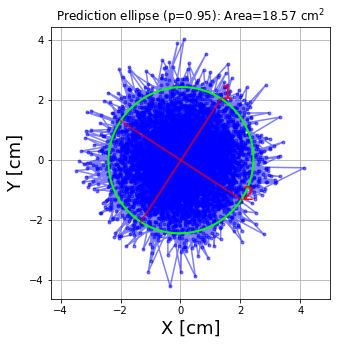Area = 18.572765377574335
Semi-axes = [2.45610669 2.40701874]
Angles = [ 56.92978672 -33.07021328]
Center = [ 0.01641617 -0.00989277]
Rotation matrix =
[[ 0.54566638  0.83800251]
[ 0.83800251 -0.54566638]]


For other data:

In :
y = np.cumsum(np.random.randn(3000)) / 50
x = np.cumsum(np.random.randn(3000)) / 100
area, axes, angles, center, R = hyperellipsoid(x, y, units='cm', show=True)
print('Area =', area)
print('Semi-axes =', axes)
print('Angles =', angles)
print('Center =', center)
print('Rotation matrix =\n', R)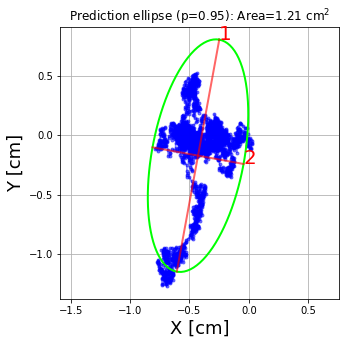Area = 1.2145303675104402
Semi-axes = [0.99253322 0.38950538]
Angles = [ 79.62776911 -10.37223089]
Center = [-0.42756993 -0.17109987]
Rotation matrix =
[[ 0.18004242  0.98365885]
[ 0.98365885 -0.18004242]]


### Find points outside the ellipse¶

The outside_hyperellipsoid function can be used to determine whether specific points lie outside the ellipse as demonstrated below.

Note that the proportion of points outside a 95% ellipse is expected to tend toward 0.05 as n increases.

In :
from hyperellipsoid import outside_hyperellipsoid

#Calculate ellipse and whether or not points lie outside:
np.random.seed(6)
P = np.random.randn(100, 2)  #points
volume, axes, angles, center, R = hyperellipsoid(P, pvalue=.95, show=False)
b = outside_hyperellipsoid(axes, angles, center, R, P) #binary hit-miss (outside ellipse?)
p = P[b] #points outside ellipse

#Plot:
fig = plt.figure(figsize=(4, 4))
ax  = fig.add_axes([0, 0, 1, 1])
ax.plot(p[:,0], p[:,1], 'ro', ms=8)  #points outside ellipse plotted as large red dots
volume, axes, angles, center, R = hyperellipsoid(P, pvalue=.95, show=True, ax=ax)

# Report:
print('Number of points outside ellipsoid: %d' %b.sum())
print('Proportion of points outside ellipsoid: %.3f' %b.mean())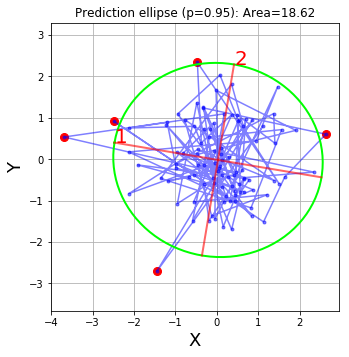Number of points outside ellipsoid: 5
Proportion of points outside ellipsoid: 0.050


#### Prediction ellipsoid¶

For a trivariate random data with normal distribution with mean 0 and variance 1, the prediction ellipsoid should be a sphere:

In :
P = np.random.randn(10000, 3)
volume, axes, angles, center, R = hyperellipsoid(P, units='cm', show=True)
print('Volume =', volume)
print('Semi-axes =', axes)
print('Angles =', angles)
print('Center =', center)
print('Rotation matrix =\n', R)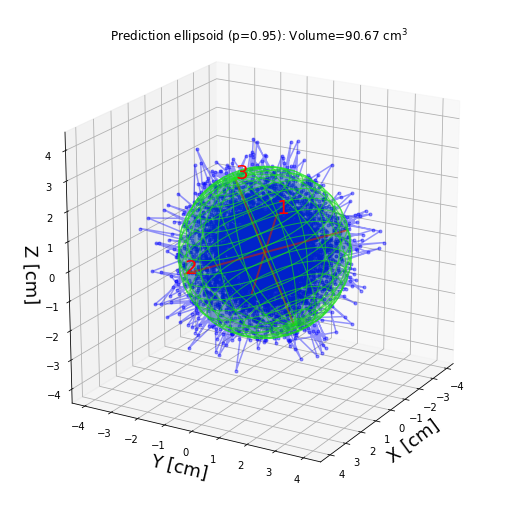Volume = 90.66797382781313
Semi-axes = [2.83080025 2.79215201 2.73852694]
Angles = [-15.88378989 -13.16031177 161.24997778]
Center = [ 0.00393022 -0.01780758  0.01150057]
Rotation matrix =
[[-0.92206064 -0.36817207  0.11938807]
[ 0.31299781 -0.89074547 -0.32955255]
[ 0.22767643 -0.26649923  0.93655838]]


For other data:

In :
P = np.random.randn(1000, 3)
P[:, 2] = P[:, 2] + P[:, 1]*.5
P[:, 1] = P[:, 1] + P[:, 0]*.5
volume, axes, angles, center, R = hyperellipsoid(P, units='cm', show=True)
print('Volume =', volume)
print('Semi-axes =', axes)
print('Angles =', angles)
print('Center =', center)
print('Rotation matrix =\n', R)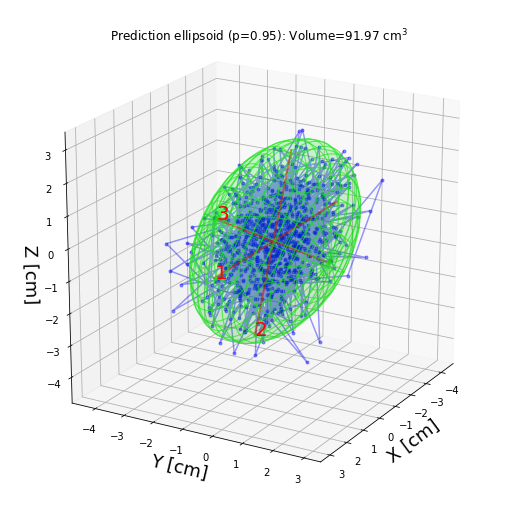Volume = 91.97004935222222
Semi-axes = [3.85939857 3.00260608 1.89469722]
Angles = [-55.60639772 -39.13088344 118.46977354]
Center = [-0.01948494 -0.03595066 -0.00582128]
Rotation matrix =
[[-0.36977539 -0.74480946 -0.55545029]
[ 0.68189918  0.18851428 -0.70673607]
[ 0.63109402 -0.64009471  0.43817702]]


### Find points outside the (hyper)ellipsoid¶

The outside_hyperellipsoid function can be used to determine whether specific points lie outside the ellipsoid as demonstrated below.

Note that the proportion of points outside a 95% ellipsoid is expected to tend toward 0.05 as n increases.

In :
from mpl_toolkits.mplot3d import Axes3D
import matplotlib.animation as animation
from hyperellipsoid import _plot, outside_hyperellipsoid

# fig = plt.figure(figsize=(7, 7))
# ax = fig.add_axes([0, 0, 1, 1], projection='3d')

#Calculate ellipsoid and whether or not points lie outside:
np.random.seed(6)
P = np.random.randn(100, 3)  #points
volume, axes, angles, center, R = hyperellipsoid(P, pvalue=.95, show=False)
b = outside_hyperellipsoid(axes, angles, center, R, P) #binary hit-miss (outside ellipsoid?)
p = P[b] #points outside ellipsoid

#Plot:
fig = plt.figure(figsize=(7, 7))
ax  = fig.add_axes([0, 0, 1, 1], projection='3d')
ax.plot(p[:,0], p[:,1], p[:,2], 'ro', ms=8)  #points outside ellipse plotted as large red dots
volume, axes, angles, center, R = hyperellipsoid(P, pvalue=.95, show=True, ax=ax)

# Report:
print('Number of points outside ellipsoid: %d' %b.sum())
print('Proportion of points outside ellipsoid: %.3f' %b.mean())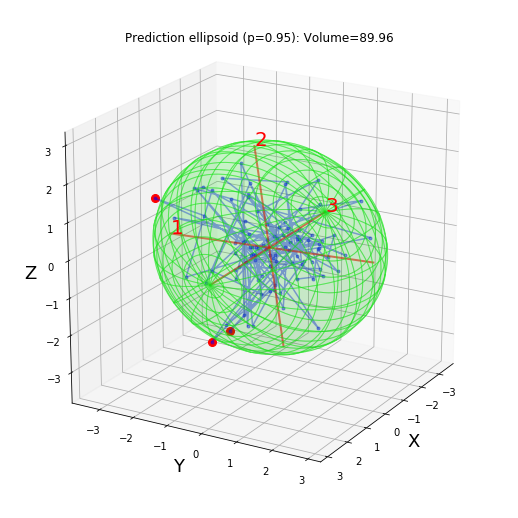Number of points outside ellipsoid: 3
Proportion of points outside ellipsoid: 0.030


To be able to rotate and zoom the figure, for now you will have to plot it in a separate window:

In :
# plot figure in a separate window
%matplotlib qt

In :
hyperellipsoid(P, units='cm', show=True);